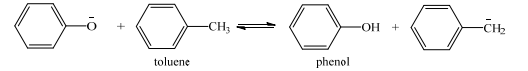# Problem: Consider the following reaction given below. One of the 5 resonance structures (Lewis structures) that contribute to the resonance hybrid of the conjugate base of phenol is represented (below) as one of the products in the given chemical equation. (a) Draw the additional 4 different resonance structures (Lewis structures) that contribute to the resonance hybrid of the conjugate base of toluene. For each of the four Lewis structures (resonance structures) that you give, you must show all atoms, all bonding valence electrons, all unshared valence electrons, and each nonzero formal charge. (b) Clearly give the numerical value (in degrees) for the HCH bond angle in the conjugate base of toluene. (c) The equilibrium shown below lies to the left. This, of course, means that phenol is more acidic than toluene. Using structural considerations, provide a clear, concise explanation (but in as much detail as possible) why this equilibrium lies to the left. NOTE that a correct answer to this part is not that “the pKa of phenol is less than the pKa of toluene” because this is merely a restatement of the fact that phenol is more acidic than toluene.

###### FREE Expert Solution
81% (403 ratings)
###### Problem Details

Consider the following reaction given below. One of the 5 resonance structures (Lewis structures) that contribute to the resonance hybrid of the conjugate base of phenol is represented (below) as one of the products in the given chemical equation. (a) Draw the additional 4 different resonance structures (Lewis structures) that contribute to the resonance hybrid of the conjugate base of toluene. For each of the four Lewis structures (resonance structures) that you give, you must show all atoms, all bonding valence electrons, all unshared valence electrons, and each nonzero formal charge. (b) Clearly give the numerical value (in degrees) for the HCH bond angle in the conjugate base of toluene. (c) The equilibrium shown below lies to the left. This, of course, means that phenol is more acidic than toluene. Using structural considerations, provide a clear, concise explanation (but in as much detail as possible) why this equilibrium lies to the left. NOTE that a correct answer to this part is not that “the pKa of phenol is less than the pKa of toluene” because this is merely a restatement of the fact that phenol is more acidic than toluene.